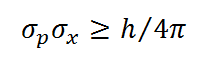# Heisenberg's Uncertainty Principle Calculator

This is a CalcTown calculator to calculate the minimum uncertainty in momentum/position or Energy/time or angular momentum/angular position.

* Please enter value of uncertainty in the variable and minimum uncertainty in its complementary variable is given as output.

SI Units:

Position -m, Linear Momentum-kg-m/s

Energy-J ,Time-s

Angular momentum-kg-m2/s, Angular position-rad

#### Resultwhere
σp is the uncertainty in one variable. and

σx is the uncertainty in its complementary variable

* Please note that Heisenberg's uncertainty principle is true for many complementary variables, like Linear Momentum-Position, Energy-Time, Angular Momentum-Angular position etc.